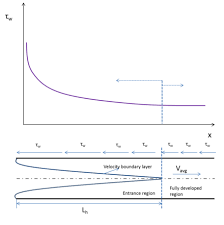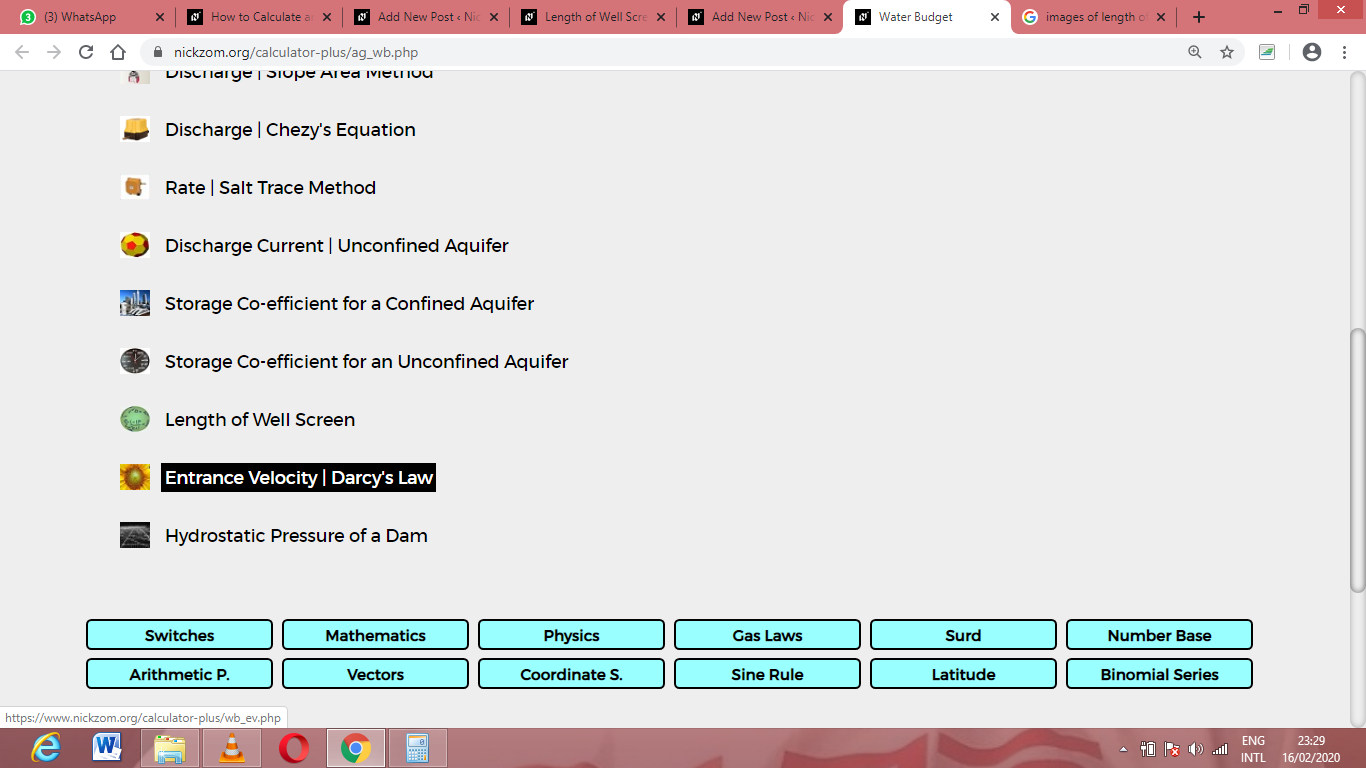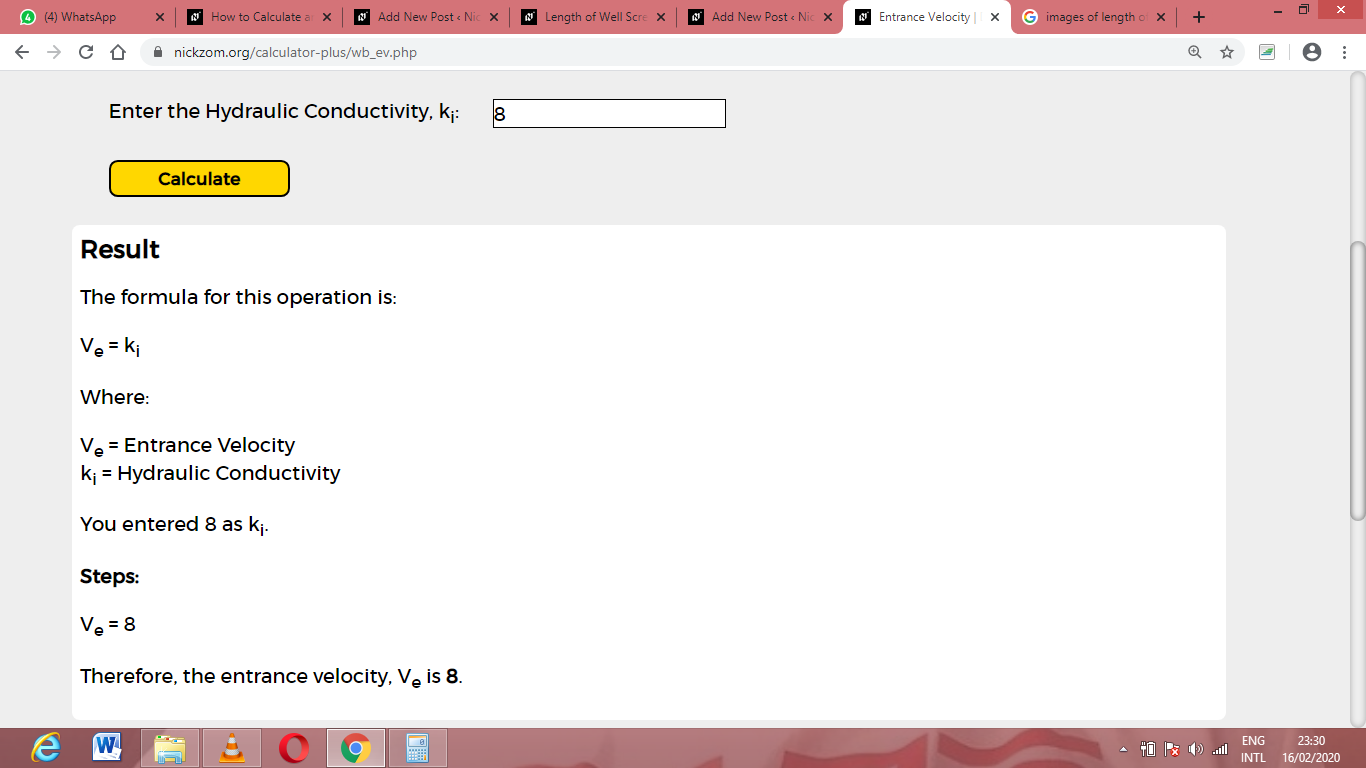# How to Calculate and Solve for Entrance Velocity | Darcy’s Law | Water BudgetThe image above represents entrance velocity.

To compute for entrance velocity, one essential parameters are needed and these parameters are Hydraulic Conductivity (ki).

The formula for calculating entrance velocity:

Ve = ki

Where:

Ve = Entrance Velocity
ki = Hydraulic Conductivity

Let’s solve an example;
Find the entrance velocity when the hydraulic conductivity is 8.\

This implies that;

ki = Hydraulic Conductivity = 8

Ve = ki
Ve = 8

Therefore, the entrance velocity is 8.

Nickzom Calculator – The Calculator Encyclopedia is capable of calculating the entrance velocity.

To get the answer and workings of the entrance velocity using the Nickzom Calculator – The Calculator Encyclopedia. First, you need to obtain the app.

You can get this app via any of these means:

To get access to the professional version via web, you need to register and subscribe for NGN 1,500 per annum to have utter access to all functionalities.
You can also try the demo version via https://www.nickzom.org/calculator

Apple (Paid) – https://itunes.apple.com/us/app/nickzom-calculator/id1331162702?mt=8
Once, you have obtained the calculator encyclopedia app, proceed to the Calculator Map, then click on Agricultural under Engineering.Now, Click on Water Budget under AgriculturalNow, Click on Entrance Velocity under Water BudgetThe screenshot below displays the page or activity to enter your values, to get the answer for the entrance velocity according to the respective parameters which are the Hydraulic Conductivity (ki).Now, enter the values appropriately and accordingly for the parameters as required by the Hydraulic Conductivity (ki) is 8.Finally, Click on CalculateAs you can see from the screenshot above, Nickzom Calculator– The Calculator Encyclopedia solves for the entrance velocity and presents the formula, workings and steps too.